Learning Library
Who we are
Guidance

# 5.MD.C.4 Worksheets, Workbooks, Lesson Plans, and Games

#### CCSS.MATH.CONTENT.5.MD.C.4

:
"Measure volumes by counting unit cubes, using cubic cm, cubic in, cubic ft, and improvised units."

These worksheets can help students practice this Common Core State Standards skill.

## Worksheets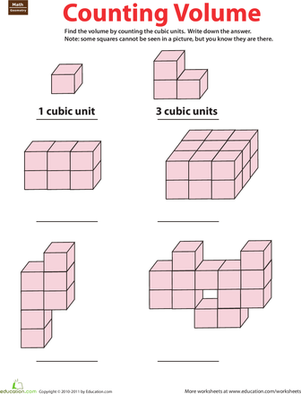Geometry: Counting Volume
Worksheet
Geometry: Counting Volume
Is your third-grader ready to start learning about volume? Introduce them to the concept with this clear and easily understood worksheet.
Math
Worksheet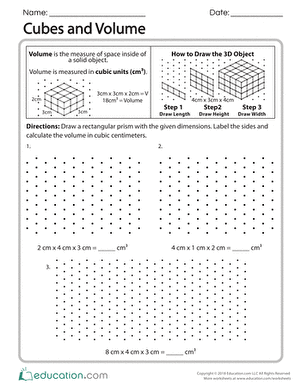Cubes and Volume
Worksheet
Cubes and Volume
This worksheet will challenge students’ understanding of volume and the equation l x w x h = V. Then, once they solve the equation have them draw the 3-D objects. The objects will all be rectangular prisms.
Math
Worksheet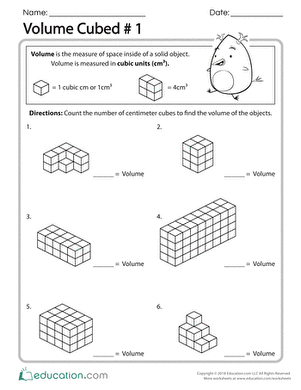Volume Cubed #1
Worksheet
Volume Cubed #1
Ready for your fifth graders to take their learning of cubic units to the next level? With this worksheet, students will count the stacked centimeter blocks to determine the volume of the solid object.
Math
Worksheet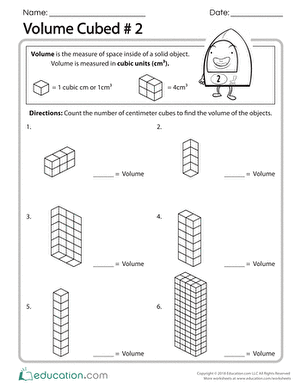Volume Cubed #2
Worksheet
Volume Cubed #2
With this worksheet, your students will take their learning of centimeter cubes to a 2-D rendering of their work. Students will count the centimeter blocks to determine the volume of the solid object.
Math
Worksheet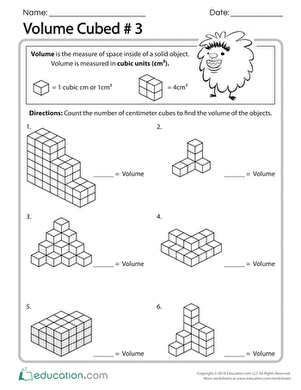Volume Cubed #3
Worksheet
Volume Cubed #3
Centimeter cubes have gone wild with these irregular shapes! Students will be challenged to calculate the volume of different shapes by counting the cubes.
Math
Worksheet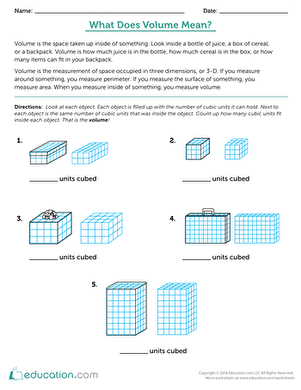What Does Volume Mean?
Worksheet
What Does Volume Mean?
In this activity, compare the volume of the container with the volume of its contents to calculate how much each can hold.
Math
Worksheet

## Lesson Plans

No plans found for this common core node.

## Workbooks

No workbooks found for this common core node.

## Games

No games found for this common core node.

## Exercises

No exercises found for this common core node.

What could we do to improve Education.com?

Please note: Use the Contact Us link at the bottom of our website for account-specific questions or issues.

What would make you love Education.com?